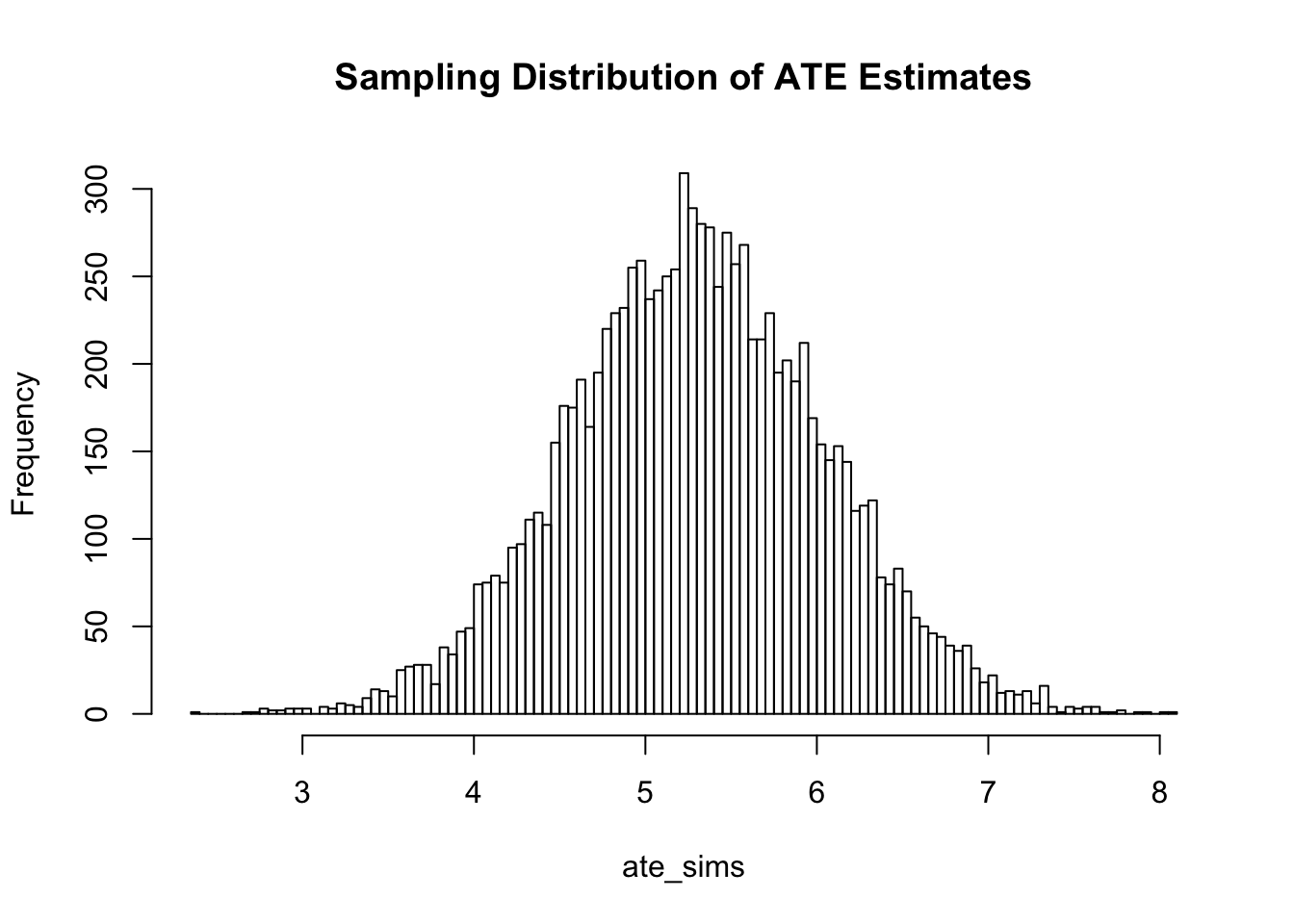Lets compare the coverage of two confidence intervals - one made using Normal approximation (equation 3.6 or HC2 robust standard errors), the other under the constant treatment effects assumption.

# Create Dataset

library(dplyr)
library(randomizr)
library(knitr)

set.seed(100)

data <- data_frame(Y0 = rnorm(500, mean = 0, sd = 8), Y1 = Y0 + rnorm(500, mean = 5,
sd = 4), X1 = as.numeric(Y0 >= 0), X2 = randomizr::complete_ra(N = 500,
m = 250), C1 = ifelse(Y0 <= -1, 1, ifelse((Y0 > -1) & (Y0 <= 0), 2, ifelse((Y0 >
0) & (Y0 <= 1), 3, 4))), C2 = sample(x = c(1, 2, 3, 4), size = 500, replace = T,
prob = c(0.25, 0.25, 0.25, 0.25)))

kable(head(data), caption = "Dataset for Confidence Interval Converage Test",
row.names = T)
Dataset for Confidence Interval Converage Test
Y0 Y1 X1 X2 C1 C2
1 -4.0175388 -4.802689 0 1 1 2
2 1.0522493 7.315672 1 1 4 1
3 -0.6313367 2.997673 0 1 2 1
4 7.0942785 4.368866 1 0 4 2
5 0.9357702 6.907054 1 1 3 4
6 2.5490407 6.097969 1 1 4 4
true_ate <- mean(data$Y1 - data$Y0)  # True ATE
true_ate
##  5.28487

# Sampling distribution of ATE estimates

declaration <- declare_ra(N = nrow(data), m = 250)  # Complete random assignment, m=250, N=500

Z <- conduct_ra(declaration)

# Get the sampling distribution of all ATE estimates

D <- obtain_permutation_matrix(declaration)

ate_sims <- rep(NA, ncol(D))

for (i in 1:ncol(D)) {
Z_temp <- D[, i]
Y <- data$Y1 * Z_temp + data$Y0 * (1 - Z_temp)
ate_sims[i] <- mean(Y[Z_temp == 1]) - mean(Y[Z_temp == 0])
}

hist(ate_sims, breaks = 100, main = "Sampling Distribution of ATE Estimates")# Performance of Normal approximation confidence intervals

To get the coverage of confidence intervals constructed using equation 3.6 I run a simulation in which for different samples of the population ($$N_{pop}=500$$ and $$n_{sample}=200$$), I compute the ate estimate, standard error estimate, and check if the resulting confidence interval contains the true ATE (which is known from the above dataset: $$E[\tau_i] = 5.28487$$).

library(commarobust)

# Define output vector
coverage_normal <- rep(NA, 1000)
# For a random sample, create a declaration in randomizr
sample_declaration <- declare_rs(N = 500, n = 200)

for (i in 1:1000) {
# Generate a random sample
data$selected <- draw_rs(sample_declaration) sample <- data %>% filter(selected == 1) # Conduct complete random assignment Z_temp <- complete_ra(N = 200, m = 100) # Switching equation Y <- sample$Y1 * Z_temp + sample$Y0 * (1 - Z_temp) model <- lm(Y ~ Z_temp) # ATE estimate ate_estimate <- commarobust(model)[2, 1] # SE estimate (HC2 robust SE) se_estimate <- commarobust(model)[2, 2] # Construct CI ci_lower <- ate_estimate - 1.96 * se_estimate ci_upper <- ate_estimate + 1.96 * se_estimate # Check if this CI contains true ate coverage_normal[i] <- as.numeric((true_ate >= ci_lower) & (true_ate <= ci_upper)) } # Coverage of CI mean(coverage_normal) ##  0.951 # Performance of Gerber and Green SEs To get the coverage of confidence intervals constructed under the constant treatment effects assumption, I run a simulation in which for different samples ($$n_{sample} = 200$$) of the population ($$N_{pop} = 500$$), I compute the ate estimate, use that to get a hypothetical schedule of potential outcomes under the fixed effects assumption, and check if the resulting confidence interval contains the true ATE (5.28487) coverage_gerbgreen <- rep(NA, 1000) sample_declaration <- declare_rs(N = 500, n = 200) set.seed(32) for (i in 1:1000) { # Generate a random sample data$selected <- draw_rs(sample_declaration)
sample <- data %>% filter(selected == 1)
# Conduct complete random assignment
Z_temp <- complete_ra(N = 200, m = 100)
# Switching equation
Y <- sample$Y1 * Z_temp + sample$Y0 * (1 - Z_temp)
model <- lm(Y ~ Z_temp)
# ATE estimate
ate_estimate <- commarobust(model)[2, 1]
# SE estimate (under constant effects) Schedule of potential outcomes, under
# fixed effects
Y0_temp <- Y - ate_estimate * Z_temp
Y1_temp <- Y0_temp + ate_estimate
# Create permutation matrix
declaration <- declare_ra(N = length(Y), m = length(Y)/2)
D <- obtain_permutation_matrix(declaration, maximum_permutations = 1000)
# Loop to get sampling distribution
ate_sims <- rep(NA, ncol(D))
for (j in 1:ncol(D)) {
z_sim <- D[, j]
# Compute ATE
ate_sims[j] <- mean(Y1_temp[z_sim == 1]) - mean(Y0_temp[z_sim == 0])
}
# Construct CI
ci_lower <- quantile(ate_sims, probs = 0.025)
ci_upper <- quantile(ate_sims, probs = 0.975)
# Check if this CI contains true ate
coverage_gerbgreen[i] <- as.numeric((true_ate >= ci_lower) & (true_ate <=
ci_upper))
}

# Coverage of CI
mean(coverage_gerbgreen)
##  0.956

# Small and large sample properties

I write a function that computes coverage for different sample sizes: $$n_{sample} \in \{10,50,100,250\}$$.

Sample.Size Coverage.NormalApprox Coverage.GerberGreen
10 0.912 0.896
50 0.944 0.932
100 0.961 0.951
250 0.949 0.947